This page discusses methods of interfacing passive crossover circuits and real drivers for the Virtual Crossover.

To accurately predict the system response to a particular set of passive crossover circuits, realistic models of the drivers must be included. There are two basic requirements for this. First, the complex input impedance of the driver must be measured, so that you know how the crossover circuit is terminated. And secondly, the driver impulse response must be measured, in order to determine what the driver output will be for a particular crossover circuit. The Virtual Crossover can be used to carry out both these measurements.

Figure 1 illustrates the overall method of coupling the crossover circuit and the driver, and of predicting the driver output: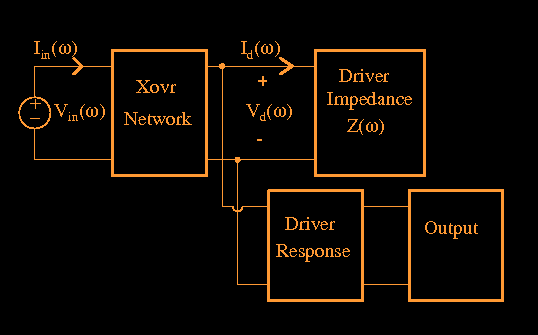Figure 1: General method of coupling passive crossover circuit model and driver.

In the figure,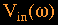is the input voltage to the crossover circuit and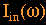is the current entering the circuit.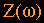is the complex input impedance of the driver; since it is given in the frequency domain, it is most convenient to solve this circuit in the frequency domain. This can be done with the IMPRSPZ.C program, ifis specified as the terminating impedance. The way IMPRSPZ.C works is as follows. At each frequency, it is initially assumed that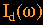, the current into the driver, is unity. Then the initial voltage across the driver is just. IMPRSPZ.C sets these conditions and solves the circuit back to the Vin terminals. But Vin is known both in the time and frequency domain, it might be for example the equal-ripple lowpass filter discussed on the page about issues in numerical modeling of circuits for the Virtual Crossover. So IMPRSPZ.C compares what it calculates at the Vin terminals to thethat it knows is the right answer. Of course in general the values will not be the same, but if the circuit is linear, the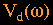value can just be scaled so that we obtain the correct. In this manner, by going through all the discrete frequencies and scaling to obtain the correct, we finally end up with the correctat the driver input terminals. And onceis known, it can be convolved with the measured driver impulse response to obtain the predicted driver output, as indicated by the lower two boxes in Fig.(1).

As an example, I will present the results of this method for two Dynaudio D76-af midranges in series that I used in one of my speaker systems. Figure 2 shows a portion of the measured impedance magnitude, out to 10kHz, for the drivers: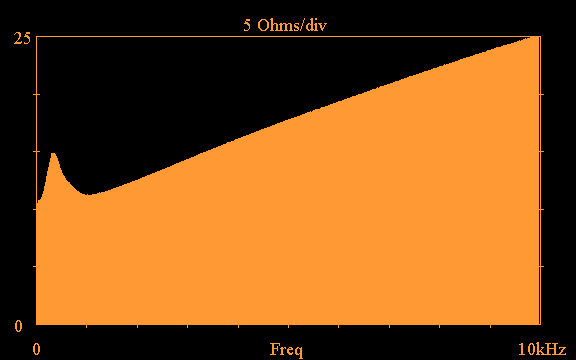Figure 2: Input impedance magnitude of two Dynaudio D76-af drivers in series, out to 10kHz.

Since we require complex impedance to characterize the drivers, this impedance also has an associated phase response, which is shown in Figure 3: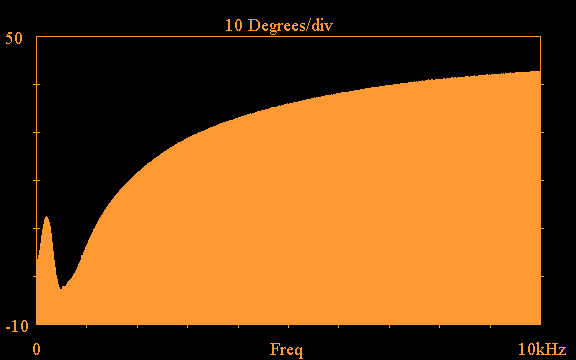Figure 2: Impedance phase angle of two Dynaudio D76-af drivers in series, out to 10kHz.

Clearly these drivers do not present anything close to a constant resistance to the crossover circuit, and it is necessary to take into account the driver impedance in order to accurately predict the crossover response.

This can be done in different ways, however. One way is to directly terminate the crossover circuit with theof Figs.(1) and (2), and to use IMPRSPZ.C to calculateat the driver terminals. This method is fine if you just want to observe the basic response of the drivers to a crossover circuit. However, the Virtual Crossover also allows you to loadas a filter for the drivers and play music, essentially simulating the passive crossover circuit in real time; in that case, the low-level noise that comes about by measuringcan be a problem.

To get around this noise issue, we can first design an impedance compensation network so that the impedance presented by the drivers is nearly a constant resistance. Then, to a very good approximation, we can assume thatin Fig.(1) can be represented by just a resistor. Then we can carry out either a time-domain or frequency-domain calculation of the crossover filter, without incurring any of the noise brought about by the impedance measurement process.

For example, the following impedance compensation circuit (RZ and CZ) causes the D76-af midrange drivers to present nearly a constant resistance for frequencies above the driver resonance: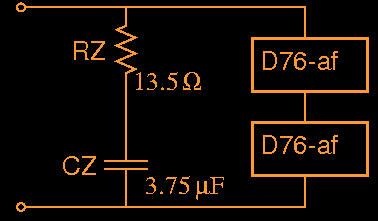Figure 3: Impedance compensation network for two D76-af midranges in series.

I offer a utility called ZOBEL.C which reads in a measured complex driver impedanceas well as an impedance compensation circuit (RZ and CZ in this case), and outputs the impedance as transformed by the compensation network. If I run ZOBEL.C, read in the complexof Figs.(2) and (3), and the RZ, CZ values of Fig.(3), the following transformed impedance magnitude results: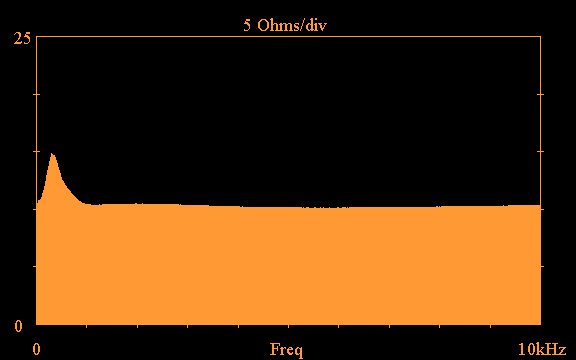Figure 4: D76-af impedance magnitude after compensation by the network in Fig.(3).

As can be seen in Fig.(4), the impedance compensation network is effective above about 1kHz, however it does not remove the impedance peak at the driver resonant frequency. This peak at driver resonance can be handled in different ways. First, we could expand the impedance compensation network of Fig.(3) to include an R-L-C branch in parallel with RZ and CZ, which would flatten the impedance peak at resonance. However, there may be situations where either the impedance peak does not have much of an effect on the crossover performance and therefore is not worth eliminating, or in some cases the peak may even be desirable, for example if it helps to flatten the combined woofer+midrange response. If it is decided to retain this impedance peak at driver resonance, it can be included as a parallel RLC network in the crossover circuit itself.

To clarify these ideas, here is a actual midrange crossover circuit that I used to obtain a filter for use in the Virtual Crossover, for the two D76-af midranges in series: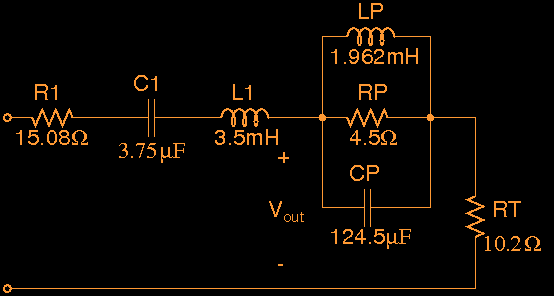Figure 5: Crossover circuit used to obtain Virtual Crossover filter for two D76-af midranges in series.

In Fig.(5), L1 and C1 perform the basic bandpass function for a first-order midrange crossover. R1 serves to set the midrange level (R1 also includes the series resistance of coil L1). RT represents the constant resistance presented by the midrange drivers above resonance, derived from the flat portion of the curve in Fig.(4). And the RP-LP-CP network puts in the impedance peak at the driver resonant frequency; RP introduces an additional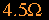at 322 Hz, and LP,CP are chosen to reproduce the proper width of the resonance.

Note that the output from Fig.(5), Vout, is taken before the RP-LP-CP network; this is because the impedance peak at resonance is actually a part of the driver, not of the crossover circuit. In my crossover I decided not to eliminate the impedance peak at resonance, because it seemed to compensate for a dip in the combined response of all the drivers.

Using either IMPRSP.C to carry out a time-domain simulation of the circuit in Fig.(5), or using IMPRSPZ.C and specifying a file with constant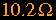terminating impedance, here is the result for the waveform at the Vout terminals of Fig.(5), where a 30kHz equal-ripple low-pass filter was impressed at the input: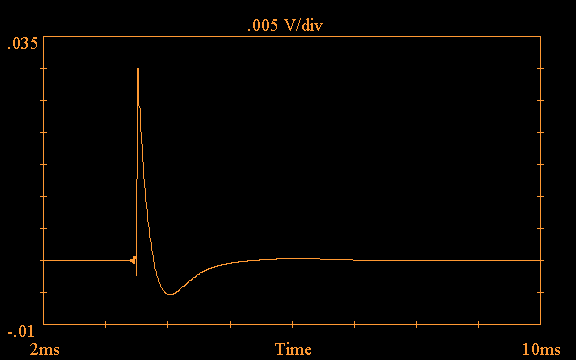Figure 6: Portion of the time-domain response at the Vout terminals of Fig.(5), with a 30kHz equal-ripple lowpass-filter at the input.

Fig.(6) is the waveform (or a judiciously chosen portion of it) that would be loaded into the Virtual Crossover as the midrange crossover filter.

Taking the FFT of the time-domain waveform of Fig.(6), we obtain the following frequency response for the midrange crossover filter: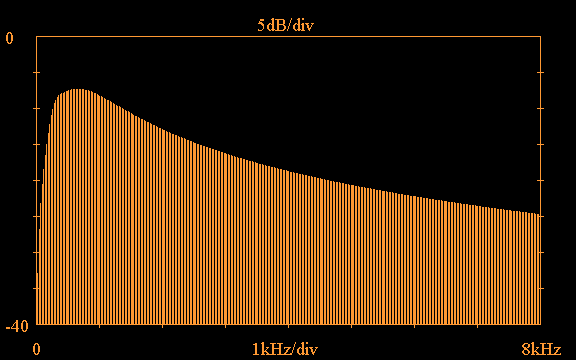Figure 7: Frequency response magnitude of the midrange crossover filter of Fig.(6) out to 8kHz.

This is the type of bandpass characteristic that you would expect for a midrange that is to be crossed over both to a woofer on the low end and a tweeter on the high end. The effect of the driver impedance peak can be seen as a slight bump in the curve around 322 Hz.

The waveform in Fig.(7) is fine as far as telling us what the response of the midrange crossover circuit is. However, it doesn't tell us what the response of the midrange drivers will be, when they are fed by this crossover filter. Referring back to Fig.(1), what we have so far determined isin that figure. To determine the actual response of the midrange drivers, we could pursue one of two routes at this point: we could either load the filter of Fig.(6) into the Virtual Crossover and carry out a measurement of the driver response through the crossover filter; or, if we have previously measured the impulse response of the midrange drivers, we could convolve the crossover filter response of Figs.(6) and (7) with the measured driver impulse response. Here I will show an example of the latter procedure. Let's say we have used the Virtual Crossover to obtain the impulse response of the D76-af midrange drivers mounted in the speaker enclosure; in my case the following result is obtained:Figure 8: Portion of impulse response of D76-af midrange drivers mounted in the final speaker enclosure as measured by the Virtual Crossover.

Fig.(8) was obtained using an MLS sequence as the measurement source. Normally when you carry out a driver impulse response measurement, unless you happen to have access to an anechoic chamber, you will want to carry out a "quasi-anechoic" measurement, which means that you truncate the resulting waveform at some point in time, hopefully thereby separating the room reflections from the primary speaker response. Sometimes it is clear how to truncate the waveform, but other times, especially for woofers, it is not clear at all. The vertical line in Fig.(8) is my best guess as to where this demarcation should be. If all the data to the right of the vertical line in Fig.(8) is treated as zero, the following is obtained for the frequency response magnitude of the midrange drivers mounted in the speaker enclosure:Figure 9: Magnitude of the frequency response of D76-af midrange drivers mounted in speaker enclosure out to 8kHz, based on the measured data of Fig.(8).

The main feature of this response is the pronounced dip around 2.5kHz, which I am guessing is due to the interaction of the midrange drivers with the speaker cabinet, since isolated D76-af drivers do not exhibit such a dip.

The final step in obtaining the midrange drivers' response from the crossover of Fig.(5) is to convolve the driver impulse response of Figs.(8) and (9) with the crossover circuit response of Figs.(6) and (7). I wrote a program called XOVR.C which reads in both a crossover filter response and a driver impulse response, and carries out the convolution (it is convolution in the time domain, but multiplication in the frequency domain) to determine the overall driver response to the crossover. In fact the XOVR.C program can go one step further, it can read such responses for all the crossover filters and drivers in the system, calculate all the individual responses, and combine them all vectorially with specified gains and time delays, to determine the complete speaker system response for a given crossover. For now I will just show the result for the midrange drivers by themselves. If I run XOVR.C and specify the driver impulse response of Fig.(8) as well as the crossover circuit response of Fig.(6), I obtain the following result in the time domain: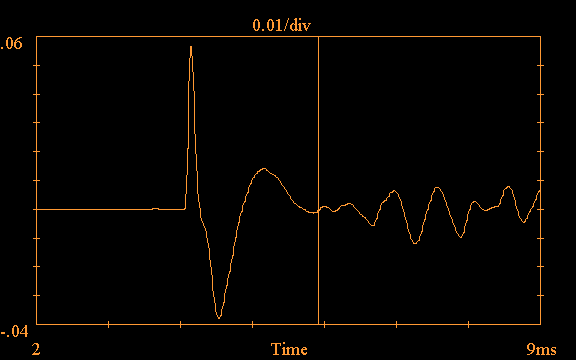Figure 10: Result of convolving the midrange crossover circuit response of Fig.(6) and the midrange driver impulse response of Fig.(8), using the XOVR.C program.

Truncating Fig.(10) at the vertical line and taking the resulting FFT yields the frequency response magnitude of the midrange drivers as follows: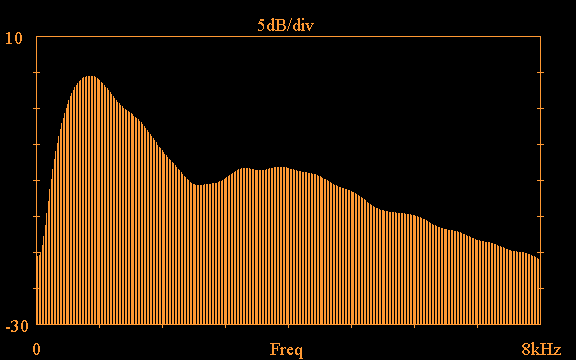Figure 11: Overall frequency response magnitude of the D76-af midrange drivers using the crossover circuit of Fig.(5).

If similar results are obtained for the other drivers in the system, they can all be combined with the XOVR.C program to obtain the overall response of the speaker system.

Home